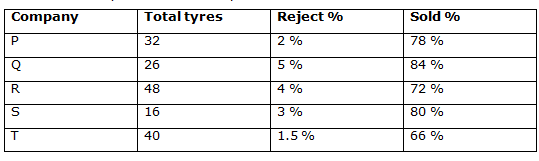# IBPS Clerk Prelims 2018 – Quantitative Aptitude Questions Day-15

Dear Readers, Bank Exam Race for the Year 2018 is already started, To enrich your preparation here we have providing new series of Practice Questions on Quantitative Aptitude – Section. Candidates those who are preparing for IBPS Clerk Prelims 2018 Exams can practice these questions daily and make your preparation effective.

[WpProQuiz 4134]

Click here to view Quantitative Aptitude Questions in Hindi

Directions (Q. 1 – 5): What approximate value should come in place of question mark (?) in the following questions?

1) 26 % of 349.75 – 32 % of 599.27 =? – 157.94 – (27.21)2

a) 820

b) 568

c) 786

d) 634

e) 912

2) 5 2/3 of 299.78 + 3 1/8 of 429.67 =? % of 749.85

a) 384

b) 360

c) 448

d) 412

e) 490

3) (553.672 + 959.325 – 432.72) ÷ 14.85 =?

a) 72

b) 86

c) 65

d) 58

e) 95

4) [((149 ÷ 3) ÷ 9.97)2 + (64.13 × 9.05)1/2] = (?)2

a) 12

b) 18

c) 7

d) 23

e) 30

5) ? ÷ 3.9 + 72.03 × 15 = 4824.12 + 1598.23

a) 25682

b) 21368

c) 18924

d) 16780

e) 27950

Directions (Q. 6 – 10) Study the following information carefully and answer the given questions.

The following table shows the total number of tyres (In lakhs) manufactured by 5 different companies in a certain year.6) Total number of tyres rejected by the company P is approximately what percentage more/less than the total number of tyres rejected by the company T?

a) 7 % less

b) 15 % more

c) 15 % less

d) 24 % more

e) 7 % more

7) Find the average number of tyres manufactured by all the given companies together?

a) 32.4 lakhs

b) 28.5 lakhs

c) 30.8 lakhs

d) 34.6 lakhs

e) None of these

8) Find the difference between the total number of tyres sold by the company Q to that of company S?

a) 792600

b) 756800

c) 833200

d) 809270

e) None of these

9) Find the ratio between the total number of tyres rejected by the company Q to that of R?

a) 72: 55

b) 53: 115

c) 84: 137

d) 65: 96

e) None of these

10) Total number of tyres sold by the company P is approximately what percentage of total number of tyres sold by the company R?

a) 60 %

b) 75 %

c) 50 %

d) 85 %

e) 105 %

Answers :

Direction (1-5) :

1) Answer: c)

26 % of 350 – 32 % of 600 = x – 158 – 272

(26/100)*350 – (32/100)*600 = x – 158 – 729

91 – 192 + 158 + 729 = x

X = 786

2) Answer: d)

6 of 300 + 3 of 430 = x% of 750

(300*6) + (3*430) = (x/100)*750

1800 + 1290 = 15x/2

(3090*2)/15 = x

X = 412

3) Answer: a)

(554 + 959 – 433)/15 = x

X = 1080/15 = 72

4) Answer: c)

[((150 ÷ 3) ÷ 10)2 + (64 × 9)1/2] = x2

(150/30)2 + (8*3) = x2

25 + 24 = x2

X2 = 49

X = 7

5) Answer: b)

x ÷ 4 + 72 × 15 = 4824 + 1598

(x/4) + 1080 = 6422

(x/4) = 5342

X = 5342*4 = 21368

Direction (6-10) :

6) Answer: e)

Total number of tyres rejected by the company P

= > 3200000*(2/100) = 64000

Total number of tyres rejected by the company T

= > 4000000*(1.5/100) = 60000

Required % = [(64000 – 60000)/60000]*100 = 7 % more

7) Answer: a)

The average number of tyres manufactured by all the given companies together

= > (32 + 26 + 48 + 16 + 40)/5

= > 162/5 = 32.4 lakhs

8) Answer: c)

The total number of tyres sold by the company Q

= >2600000*(95/100)*(84/100) = 2074800

The total number of tyres sold by the company S

= > 1600000*(97/100)*(80/100) = 1241600

Required difference = 2074800 – 1241600 = 833200

9) Answer: d)

The total number of tyres rejected by the company Q

= > 26*(5/100) = 1.3 lakhs

The total number of tyres rejected by the company R

= > 48*(4/100) = 1.92 lakhs

Required ratio = 1.3: 1.92 = 130: 192 = 65: 96

10) Answer: b)

Total number of tyres sold by the company P

= > 3200000*(98/100)*(78/100) = 2446080

Total number of tyres sold by the company R

= > 4800000*(96/100)*(72/100) = 3317760

Required % = (2446080/3317760)*100 = 73.726 = 75 %

Daily Practice Test Schedule | Good Luck

 Topic Daily Publishing Time Daily News Papers & Editorials 8.00 AM Current Affairs Quiz 9.00 AM Current Affairs Quiz (Hindi) 9.30 AM IBPS Clerk Prelims – Reasoning 10.00 AM IBPS Clerk Prelims – Reasoning (Hindi) 10.30 AM IBPS Clerk Prelims – Quantitative Aptitude 11.00 AM IBPS Clerk Prelims – Quantitative Aptitude (Hindi) 11.30 AM Vocabulary (Based on The Hindu) 12.00 PM IBPS PO Prelims – English Language 1.00 PM SSC Practice Questions (Reasoning/Quantitative aptitude) 2.00 PM IBPS PO/Clerk – GK Questions 3.00 PM SSC Practice Questions (English/General Knowledge) 4.00 PM Daily Current Affairs Updates 5.00 PM IBPS PO Mains – Reasoning 6.00 PM IBPS PO Mains – Quantitative Aptitude 7.00 PM IBPS PO Mains – English Language 8.00 PM

0 0 votes
Rating0 Comments
Inline Feedbacks
View all comments